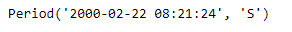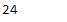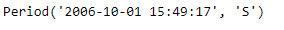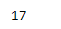Open in App
Not now

# Python | Pandas Period.second

• Last Updated : 13 Sep, 2021

Python is a great language for doing data analysis, primarily because of the fantastic ecosystem of data-centric python packages. Pandas is one of those packages and makes importing and analyzing data much easier.
Pandas Period.second attribute returns an integer value which represents the value of seconds in the given Period object.

Syntax : Period.second
Parameters : None
Return : seconds

Example #1: Use Period.second attribute to find the value of seconds present in the given Period object.

## Python3

 `# importing pandas as pd``import` `pandas as pd` `# Create the Period object``prd ``=` `pd.Period(freq ``=``'S'``, year ``=` `2000``, month ``=` `2``, day ``=` `22``,``                         ``hour ``=` `8``, minute ``=` `21``, second ``=` `24``)` `# Print the Period object``print``(prd)`

Output :Now we will use the Period.second attribute to find the number of second in the given period object.

## Python3

 `# return the number of seconds``prd.second`

Output :As we can see in the output, the Period.second attribute has returned 24 indicating that the number of seconds in prd object is 24.
Example #2: Use Period.second attribute to find the value of seconds present in the given Period object.

## Python3

 `# importing pandas as pd``import` `pandas as pd` `# Create the Period object``prd ``=` `pd.Period(freq ``=``'S'``, year ``=` `2006``, month ``=` `10``,``               ``hour ``=` `15``, minute ``=` `49``, second ``=` `17``)` `# Print the Period object``print``(prd)`

Output :Now we will use the Period.second attribute to find the number of second in the given period object.

## Python3

 `# return the number of seconds``prd.second`

Output :As we can see in the output, the Period.second attribute has returned 17 indicating that the number of seconds in prd object is 17.

My Personal Notes arrow_drop_up